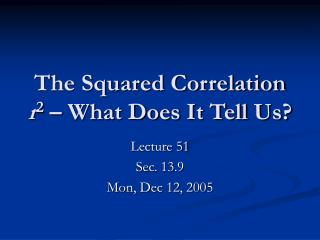Download PresentationThe Squared Correlation r 2 – What Does It Tell Us?

# The Squared Correlation r 2 – What Does It Tell Us?

Download Presentation## The Squared Correlation r 2 – What Does It Tell Us?

- - - - - - - - - - - - - - - - - - - - - - - - - - - E N D - - - - - - - - - - - - - - - - - - - - - - - - - - -
##### Presentation Transcript

1. The Squared Correlation r2 – What Does It Tell Us? Lecture 51 Sec. 13.9 Mon, Dec 12, 2005

2. Residual Sum of Squares • Recall that the line of “best” fit was that line with the smallest sum of squared residuals. • This is also called the residual sum of squares:

3. Other Sums of Squares • There are two other sums of squares associated with y. • The regression sum of squares: • The total sum of squares:

4. Other Sums of Squares • The regression sum of squares, SSR, measures the variability in y that is predicted by the model, i.e., the variability in y^. • The total sum of squares, SST, measures the observed variability in y.

5. Example – SST, SSR, and SSE • Plot the data in Example 13.14, p. 800, withy. 20 18 16 14 12 10 8 8 10 12 14 16

6. Example – SST, SSR, and SSE • The deviations of y fromy (observed). 20 18 16 14 12 10 8 8 10 12 14 16

7. Example – SST, SSR, and SSE • The deviations of y^ fromy (predicted). 20 18 16 14 12 10 8 8 10 12 14 16

8. Example – SST, SSR, and SSE • The deviations of y from y^ (residual deviations). 20 18 16 14 12 10 8 8 10 12 14 16

9. The Squared Correlation • It turns out that • It also turns out that

10. Explaining Variation • One goal of regression is to “explain” the variation in y. • For example, if x were height and y were weight, how would we explain the variation in weight? • That is, why do some people weigh more than others? • Or if x were the hours spent studying for a math test and y were the score on the test, how would we explain the variation in scores? • That is, why do some people score higher than others?

11. Explaining Variation • A certain amount of the variation in y can be explained by the variation in x. • Some people weigh more than others because they are taller. • Some people score higher on math tests because they studied more. • But that is never the full explanation. • Not all taller people weigh more. • Not everyone who studies longer scores higher.

12. Explaining Variation • High degree of correlation between x and y variation in x explains most of the variation in y. • Low degree of correlation between x and y variation in x explains only a little of the variation in y. • In other words, the amount of variation in y that is explained by the variation in x should be related to r.

13. Explaining Variation • Statisticians consider the predicted variation SSR to be the amount of variation in y (SST) that is explained by the model. • The remaining variation in y, i.e., residual variation SSE, is the amount that is not explained by the model.

14. Explaining Variation SST = SSE + SSR

15. Explaining Variation SST = SSE + SSR Total variation in y (to be explained)

16. Explaining Variation SST = SSE + SSR Total variation in y (to be explained) Variation in y that is explained by the model

17. Explaining Variation Variation in y that is unexplained by the model SST = SSE + SSR Total variation in y Variation in y that is explained by the model

18. Example – SST, SSR, and SSE • The total (observed) variation in y. 20 18 16 14 12 10 8 8 10 12 14 16

19. Example – SST, SSR, and SSE • The variation in y that is explained by the model. 20 18 16 14 12 10 8 8 10 12 14 16

20. Example – SST, SSR, and SSE • The variation in y that is not explained by the model. 20 18 16 14 12 10 8 8 10 12 14 16

21. Explaining Variation • Therefore, • r2 is the proportion of variation in y that is explained by the model and 1 – r2 is the proportion that is not explained by the model.

22. TI-83 – Calculating r2 • To calculate r2 on the TI-83, • Follow the procedure that produces the regression line and r. • In the same window, the TI-83 reports r2.

23. Let’s Do It! • Let’s Do It! 13.3, p. 819 – Oil-Change Data. • Do part (b) on the TI-83. • How much of the variation in repair costs is explained by frequency of oil change?